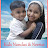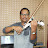How to Solve Two Step Equations | Step by Step Explanation | Algebra | MathematicsHow to Solve Two Step Equations | Step by Step Explanation | Algebra | Mathematics

How to Solve Two Step Equations | Step by Step Explanation | Algebra | Mathematics

In this video, you will learn how to solve two steps equations. The two steps involved are explained in this video. Also the method to check the solution also explained.

Amaze Tutors was established with a singular vision – to offer a high quality, international, one to-one, ‘on demand’ and truly cost effective online tutoring service.

We cover a wide range of multi-national curricula and syllabuses — including those in the Australia, UK, USA, Canada, Ireland, New Zealand, Switzerland and Middle East, CBSE, ICSE, IGCSE and IB — in a host of subject areas, including Maths, Physics, Chemistry, Biology, Science, English, etc,. We handle all grades from elementary to senior secondary levels. We also help the students in preparing for exams like Naplan, SAT, PSAT, ACT, TOEFL, IELTS, GRE, etc,.

We have professional teachers who are specialized in their respective subjects. We use best teaching methods depending upon the student’s capacity and interests. Our Services are available round-the-clock either online or on-demand.

We offer the ultimate solution for your child’s educational needs at an affordable cost. Tailor-made sessions and individual attention bring out the best from each student. We provide effective one-to-one tutoring that helps to improve your child’s grades and boost their confidence.

For more details visit www.amazetutors.com

algebra tutors,solve,equations,algebra,math,two steps,two steps equations,inverse operations,solving two step algebraic equations,algebraic equations,solve an equation,do the inverse operations,addition,subtraction,multiplication,division,checking solutions,amazetutors,solve linear equations,linear,linear equations,math tutorial,math topics,help,math help,mathematics,maths,solve by doing inverse operations,math tutoring,online tutoring,online teaching,best online tutors

algebratutors.org

1.Kids Nandus & Neerus Show says:
2.GIJU ANTYJOSE says: